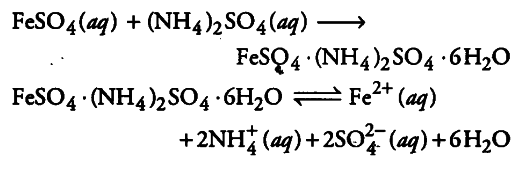# \$FeSO_{4}\$ solution mixed with solution in 1:1 molar

\$FeSO_{4}\$ solution mixed with solution in 1:1 molar ratio gives the test of
\${{Fe}^{2+}}\$ ion but \$CuSO_{4}\$ solution mixed with aqueous ammonia in 1 : 4 molar ratio does not give the test of \${{Cu}^{2+}}\$ ion. Why?

When FeSO_{4} solution are mixed in 1:1 molar ratio, Mohr’s salt (a double salt) is formed.Because {{Fe}^{2+}} ions are formed on dissolution of Mohr’s salt, its aqueous solution gives the test of {{Fe}^{2+}} ions,But when CuSO_{4} is’ mixed with ammonia, following reaction occurs.